Question

# Find the pH of the equivalence point and the volume (mL) of 0.0346 M KOH needed...

Find the pH of the equivalence point and the volume (mL) of 0.0346 M KOH needed to reach the equivalence point in the titration of 23.4 mL of 0.0390 M HNO2.

Find the pH of the two equivalence points and the volume (mL) of 0.0652 M KOH needed to reach them in the titration of 17.3 mL of 0.130 M H2CO3.

We need at least 10 more requests to produce the answer.

0 / 10 have requested this problem solution

The more requests, the faster the answer.

All students who have requested the answer will be notified once they are available.

#### Earn Coins

Coins can be redeemed for fabulous gifts.

Similar Homework Help Questions
• ### Find the pH of the equivalence point and the volume (mL) of 0.0558 M KOH needed...

Find the pH of the equivalence point and the volume (mL) of 0.0558 M KOH needed to reach the equivalence point in the titration of 23.4 mL of 0.0390 M HNO2.

• ### Be sure to answer all parts. Find the pH of the equivalence point and the volume (mL) of 0.0702 M KOH needed to reach t...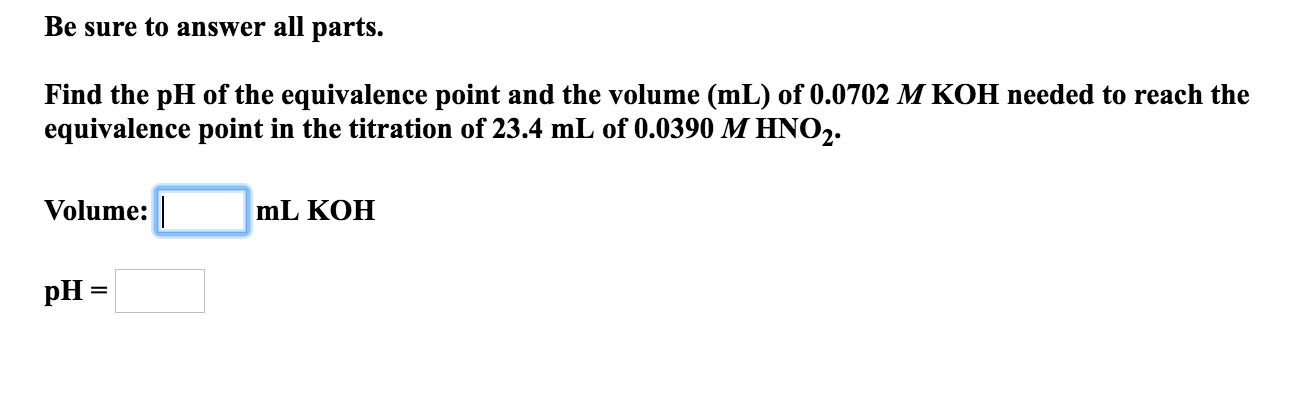Be sure to answer all parts. Find the pH of the equivalence point and the volume (mL) of 0.0702 M KOH needed to reach the equivalence point in the titration of 23.4 mL of 0.0390 M HNO,. Volume: mL KOH pH =

• ### Be sure to answer all parts. Find the pH of the equivalence point and the volume...

Be sure to answer all parts. Find the pH of the equivalence point and the volume (mL) of 0.0822 M KOH needed to reach the equivalence point in the titration of 23.4 mL of 0.0390 M HNO2. Volume: mL KOH pH =

• ### Be sure to answer all parts. Find the pH of the equivalence point and the volume...

Be sure to answer all parts. Find the pH of the equivalence point and the volume (mL) of 0.0302 M KOH needed to reach the equivalence point in the titration of 23.4 mL of 0.0390 M HNO2.

• ### Be sure to answer all parts Find the pH of the two equivalence points and the...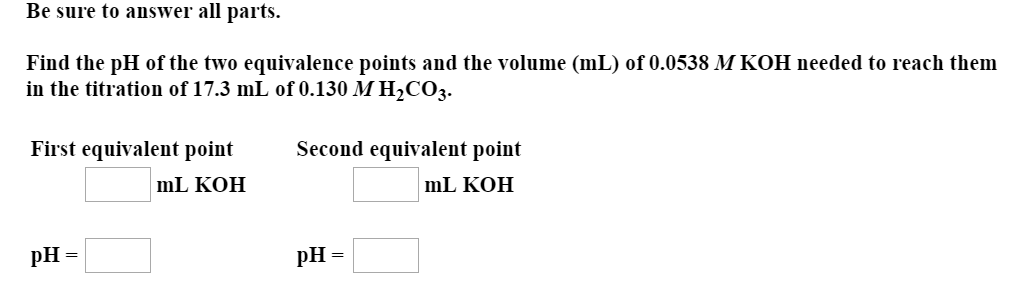Be sure to answer all parts Find the pH of the two equivalence points and the volume (mL) of 0.0538 M KOH needed to reach them in the titration of 17.3 mL of 0.130 MH2CO3. First equivalent point Second equivalent point mL KOH mL KOH pH

• ### Please show all work. Be sure to answer all parts. Find the pH of the two...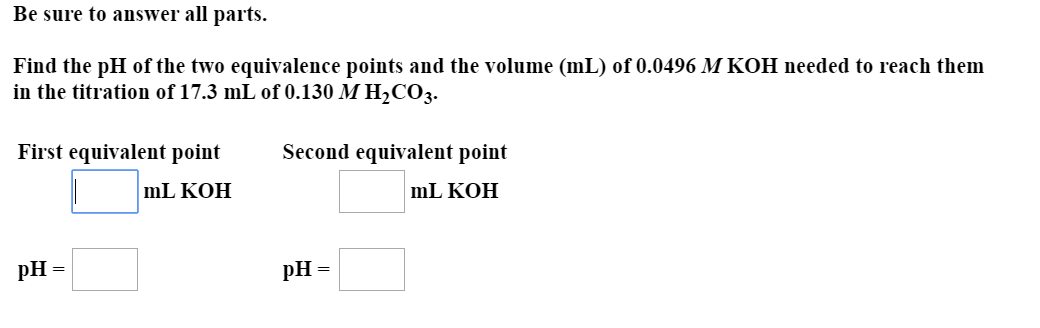Please show all work. Be sure to answer all parts. Find the pH of the two equivalence points and the volume (mL) of 0.0496 M KOH needed to reach them in the titration of 17.3 mL of 0.130 M H2CO3. Second equivalent point First equivalent point mL KOH mL KOH pH= pH =

• ### Find the pH of the equivalence point and the volume (mL) of 0.175 M HCl needed...

Find the pH of the equivalence point and the volume (mL) of 0.175 M HCl needed to reach the equivalence point in the titration of 65.5 mL of 0.234 M NH3.

• ### Find the pH of the equivalence point and the volume (mL) of 0.0372 M NaOH needed...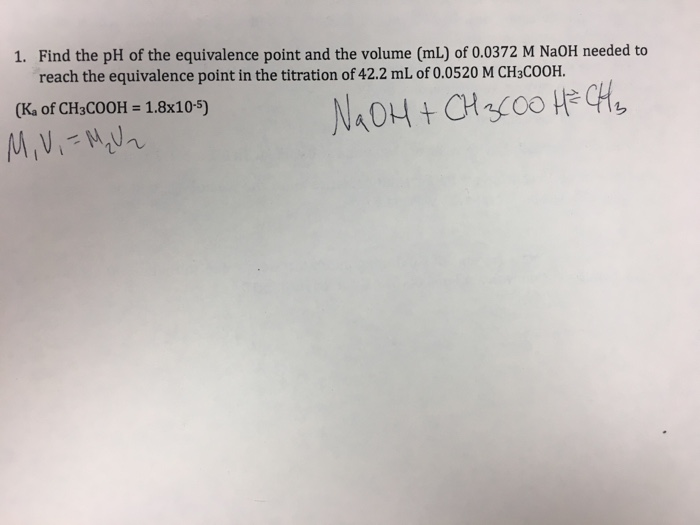Find the pH of the equivalence point and the volume (mL) of 0.0372 M NaOH needed to reach the equivalence point in the titration of 42.2 mL of 0.0520 M CH3COOH. (K_a of CH_3COOH = 1.8 times 10^-5)

• ### Find the pH of the two equivalence points and the volume (mL) of 0.527 M HNO3...

Find the pH of the two equivalence points and the volume (mL) of 0.527 M HNO3 needed to reach them in the titration of 0.188 L of 0.250 M ethylenediamine (H2NCH2CH2NH2).

• ### Find the pH of the two equivalence points and the volume (mL) of 0.367M HNO3 needed...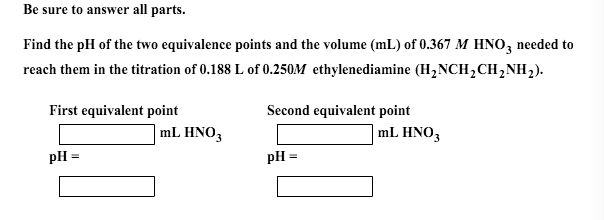Find the pH of the two equivalence points and the volume (mL) of 0.367M HNO3 needed to reach them in the titration of 0.188L of 0.250M ethylenediamine.